FFT(Fast Frequency Transform)

# FFT(Fast Frequency Transform) - Notes | Study Signals and Systems - Electronics and Communication Engineering (ECE)

 1 Crore+ students have signed up on EduRev. Have you?

UNIT:  III, FFT(Fast frequency transform)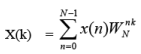0 ≤K ≤ N - 1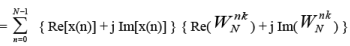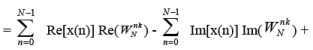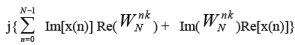• Direct evaluation of X(k) requires N 2 complex multiplications and N(N-1) complex additions.
• 4 N2 real multiplications
• { 4(N-1) + 2} N = N(4N-2) real additions
• The direct evaluation of DFT is basically inefficient because it does not use the symmetry
•  & periodicity properties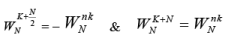DITFFT: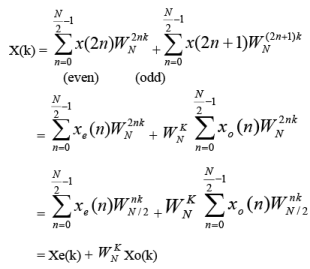Although k=0 to N-1, each of the sums are computed only for k=0 to N/2 -1, since Xe(k) & Xo(k) are periodic in k with period N/2

For K≥ N/2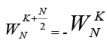X(k) for K≥N/2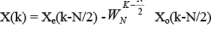N = 8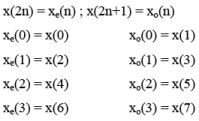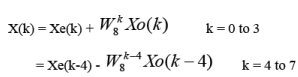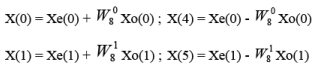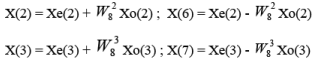X(0) & X(4) having same i/ps with opposite signs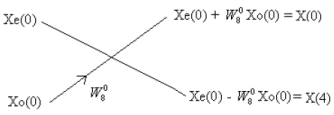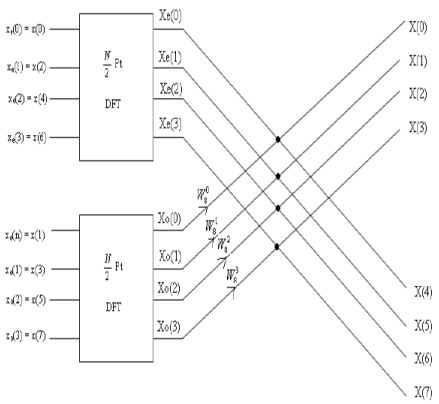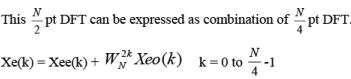(0 to 1)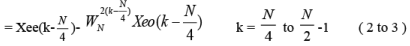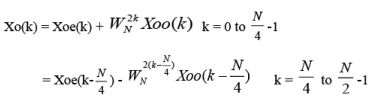For N=8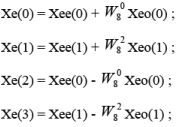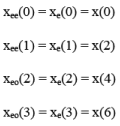Where Xee(k) is the 2 point DFT of even no. of xe(n) & Xeo(k) is the 2 point DFT of odd no. of xe(n)

Similarly, the sequence xo(n) can be divided in to even & odd numbered sequences as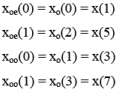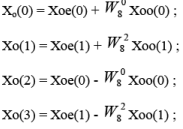Xoe(k) is the 2-pt DFT of even-numbered of xo(n)

Xoo(k) is the 2-pt DFT of odd-numbered of xo(n)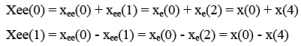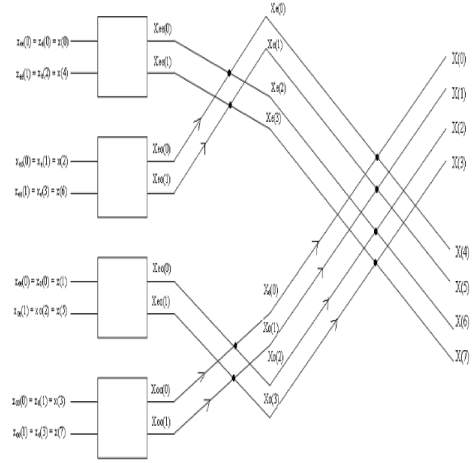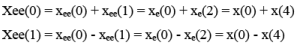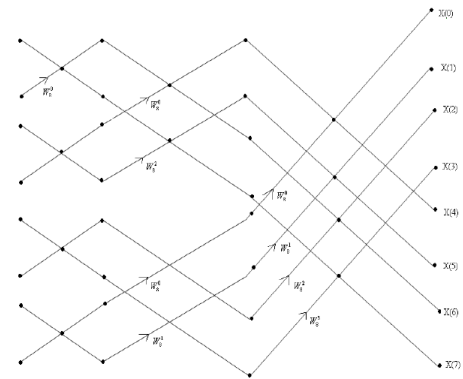No. of Stages No. of points N No. of Complex Multiplications Speed Improvement Factor: Direct N2 FFT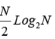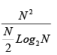2 4 16 4 4 3 8 64 12 5.33 4 16 256 32 8 5 32 1024 80 12.8 6 64 4096 192 21.33

For N=8

No of stages given by= Log2N = Log28 = 3.

No. of 2 i/p sets = 2( Log 2N  -1 ) = 4

Total No. of Complex additions using DITFFT is  NLog2N

= 8 * 3 =24

Each stage no. of butterflies in the stage= 2m-q where q = stage no. and N=2m Each butterfly operates on one pair of samples and involves two complex additions and one complex multiplication. No. of butterflies in each stage N/2

DITFFT: ( different representation) (u can follow any one) ( both representations are
correct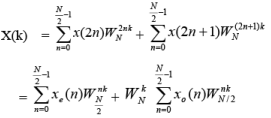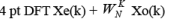k= 0 to N/2 -1 =  0 to 3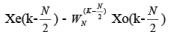k = N/2 to N-1 = 4 to 7

2 pt DFT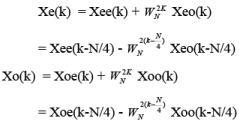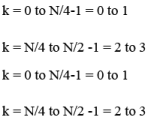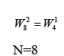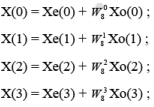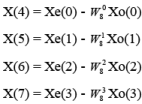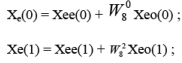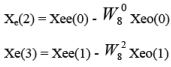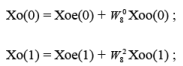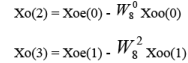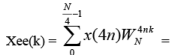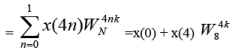Xee(0) = x(0)+x(4)

Xee(1) = x(0)-x(4)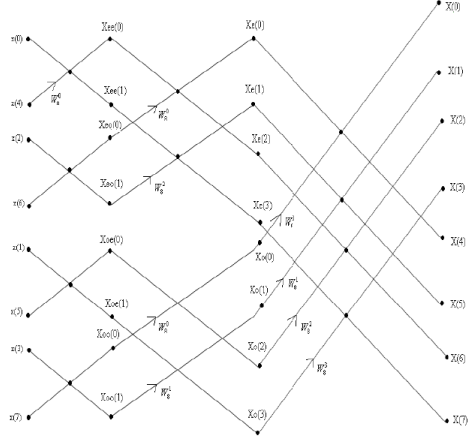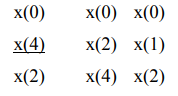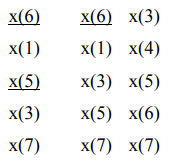Other way of representation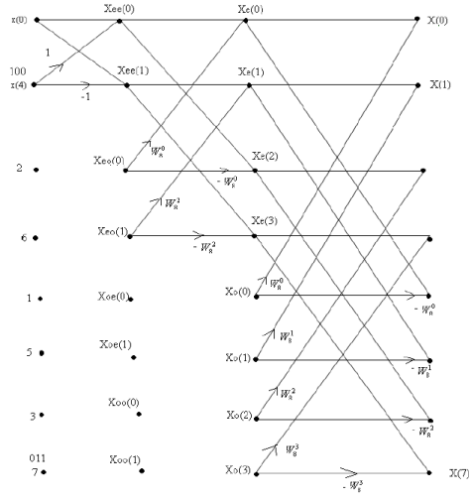DIFFFT: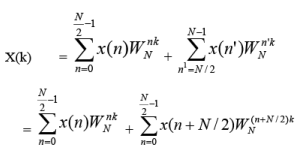put n’ = n+N/2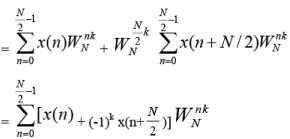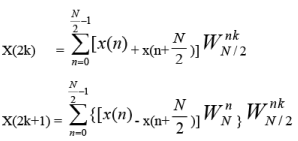Let f(n) = x(n) + x(n+N/2)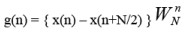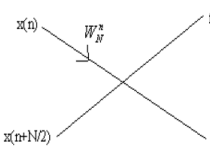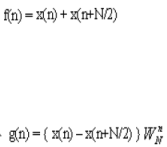N=8

f(0) = x(0) + x(4)

f(1) = x(1) + x(5)

f(2) = x(2) + x(6)

f(3) = x(3) + x(7)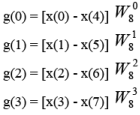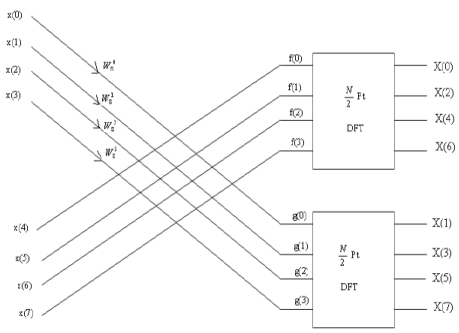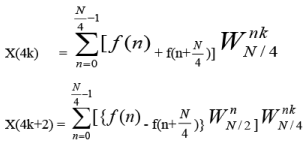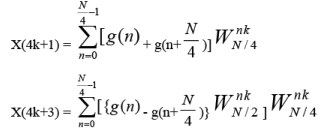X(4k) = f(0) + f(2) + [ f(1) + f(3) ]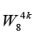X(4k+2) = f(0) – f(2) + { [ f(1) – f(3) ]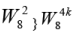X(0) = f(0) + f(2) + f(1) + f(3)

X(4) = f(0) + f(2) – [ f(1) + f(3) ]

X(2) = f(0) - f(2) + [ f(1) - f(3)] W82

X(6) = f(0) - f(2) - [ f(1) - f(3)]W82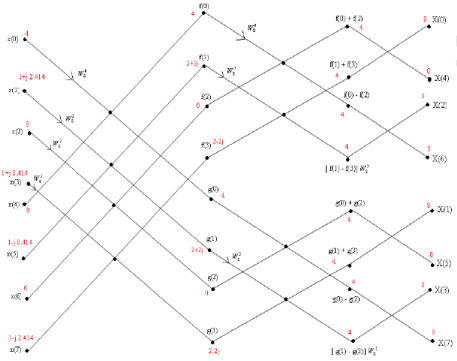Find the IDFT using DIFFFT

X(k) = { 4,  1-j 2.414,  0,  1-j 0.414,  0,  1+j 0.414,  0,  1+j 2.414 }

Out put 8x*(n) is in bit reversal order x(n) = { 1,1,1,1,0,0,0,0}

The document FFT(Fast Frequency Transform) - Notes | Study Signals and Systems - Electronics and Communication Engineering (ECE) is a part of the Electronics and Communication Engineering (ECE) Course Signals and Systems.
All you need of Electronics and Communication Engineering (ECE) at this link: Electronics and Communication Engineering (ECE)

## Signals and Systems

32 videos|76 docs|63 tests
 Use Code STAYHOME200 and get INR 200 additional OFF

## Signals and Systems

32 videos|76 docs|63 tests

Track your progress, build streaks, highlight & save important lessons and more!

,

,

,

,

,

,

,

,

,

,

,

,

,

,

,

,

,

,

,

,

,

;